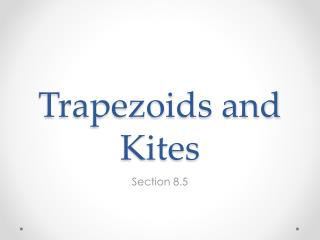DownloadDownload PresentationTrapezoids and Kites

# Trapezoids and Kites

Download Presentation## Trapezoids and Kites

- - - - - - - - - - - - - - - - - - - - - - - - - - - E N D - - - - - - - - - - - - - - - - - - - - - - - - - - -
##### Presentation Transcript

1. Trapezoids and Kites Section 8.5

2. Trapezoid Definition • A trapezoid is a quadrilateral with exactly one pair of parallel sides. These sides are the bases.

3. Is this a trapezoid?

4. Definition ofIsosceles Trapezoid • If the legs of a trapezoid are congruent, then the trapezoid is an isosceles trapezoid.

5. Theorem • If a trapezoid is isosceles, then each pair of base angles is congruent.

6. Theorem • If a trapezoid has a pair of congruent base angles, then it is an isosceles trapezoid.

7. Theorem • A trapezoid is isosceles if and only if its diagonals are congruent.

8. Midsegment Definition • The midsegment connects the midpoints of the legs.

9. Midsegment Theorem • The midsegment of a trapezoid is parallel to each base and its length is one half the sum of the bases.

10. Example:What is the length of MN in the diagram?

11. Kite Definition • A kite is a quadrilateral that has two pairs of consecutive congruent sides, but opposite sides are not congruent.

12. Theorem • If a quadrilateral is a kite, then its diagonals are perpendicular.

13. Theorem • If a quadrilateral is a kite, then exactly one pair of opposite angles are congruent.

14. Example:What is the measure of angle D in the kite below?

15. Practice • p. 546: 3-16, 18-23